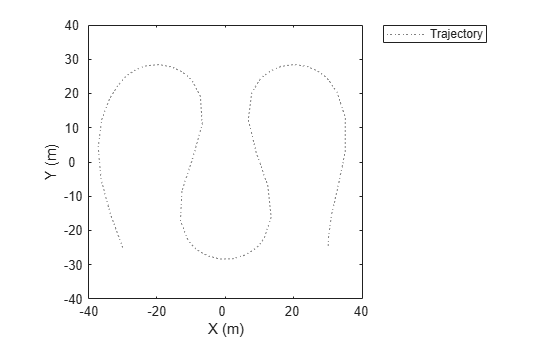# plotTrajectory

Plot set of trajectories in trajectory plotter

## Syntax

``plotTrajectory(trajPlotter,trajCoordList)``

## Description

example

````plotTrajectory(trajPlotter,trajCoordList)` specifies the trajectories to show in the trajectory plotter, `trajPlotter`. The input argument `trajCoordList` is a cell array of M-by-3 matrices, where M is the number of points in the trajectory. Each matrix in `trajCoordList` can have a different number of rows. The first, second, and third columns of each matrix correspond to the x-, y-, and z-coordinates of a curve through M points that represent the corresponding trajectory.```

## Examples

collapse all

This example shows how to create an animation of a platform moving on a trajectory.

First, create a `trackingScenario` and add waypoints for a trajectory.

```ts = trackingScenario; height = 100; d = 1; wayPoints = [ ... -30 -25 height; -30 25-d height; -30+d 25 height; -10-d 25 height; -10 25-d height; -10 -25+d height; -10+d -25 height; 10-d -25 height; 10 -25+d height; 10 25-d height; 10+d 25 height; 30-d 25 height; 30 25-d height; 30 -25+d height; 30 -25 height];```

Specify a time for each waypoint.

`elapsedTime = linspace(0,10,size(wayPoints,1));`

Next, create a platform in the tracking scenario and add trajectory information using the `trajectory` method.

```target = platform(ts); traj = waypointTrajectory('Waypoints',wayPoints,'TimeOfArrival',elapsedTime); target.Trajectory = traj;```

Record the tracking scenario to retrieve the platform's trajectory.

```r = record(ts); pposes = [r(:).Poses]; pposition = vertcat(pposes.Position);```

Create a theater plot to display the recorded trajectory.

```tp = theaterPlot('XLim',[-40 40],'YLim',[-40 40]); trajPlotter = trajectoryPlotter(tp,'DisplayName','Trajectory'); plotTrajectory(trajPlotter,{pposition})```Animate using the `platformPlotter`.

```restart(ts); trajPlotter = platformPlotter(tp,'DisplayName','Platform'); while advance(ts) p = pose(target,'true'); plotPlatform(trajPlotter, p.Position); pause(0.1) end```This animation loops through all the generated plots.## Input Arguments

collapse all

Trajectory plotter, specified as a `trajectoryPlotter` object.

Coordinates of trajectories to show, specified as a cell array of M-by-3 matrices, where M is the number of points in the trajectory. Each matrix in `trajCoordList` can have a different number of rows. The first, second, and third columns of each matrix correspond to the x-, y-, and z-coordinates of a curve through M points that represent the corresponding trajectory.

Example: ```coordList = {[1 2 3; 4 5 6; 7,8,9];[4 2 1; 4 3 1];[4 4 4; 3 1 2; 9 9 9; 1 0 2]}``` specifies three different trajectories.